# Introduction to Graphs Class 8 Notes

In this page we will explain the topics for the chapter 15 of Introduction to Graphs Class 8 Maths.We have given quality Introduction to Graphs Class 8 Notes along with video to explain various things so that students can benefits from it and learn maths in a fun and easy manner, Hope you like them and do not forget to like , social share and comment at the end of the page.
Table Of content
• Graphs
• Various type of Common Graphs
• Solved Examples on Graphs
• Cartesian system

• ## Graphs

Graphs are visual representations of data collected. They often helped us in showing the trend of the data. They are used in various field and it is of immense help to the people. There are many kind of Graph and each of these serve specific purpose.

## Various type of Common Graphs

### Bar Graph

A bar graph is used to show comparison among categories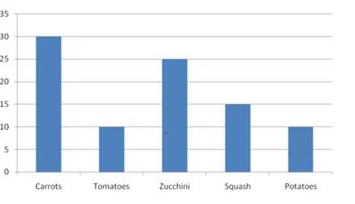### Pie Chart

A circle graph shows the relationship between a whole and its part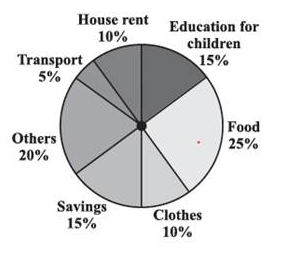### Histograms

A Histogram is a bar graph that shows data in intervals. It has adjacent bars over the intervals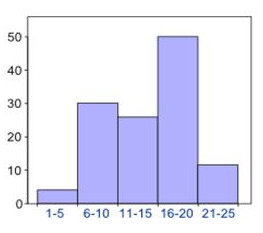### line graph

A line graph displays data that changes continuously over periods of time.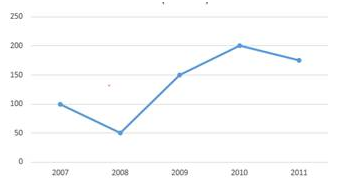### linear graph.

A line graph which is a whole unbroken line is called a linear graph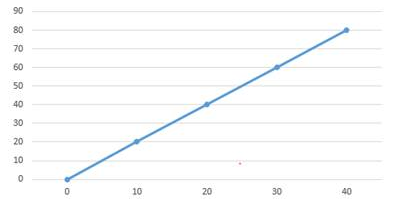## Solved Examples on Graphs

Draw the Line Graph for the following
a)
 Sales (crore) 10 15 20 15 25 Year 2013 2014 2015 2016 2017

b)

 Time 10 11 12 13 14 Temperature 250C 260C 300C 350C 400C

Solution
a)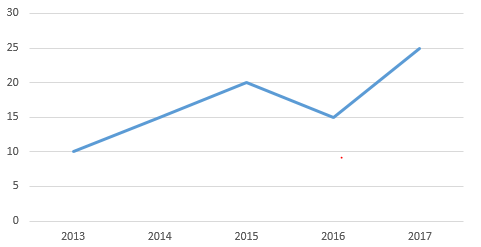b)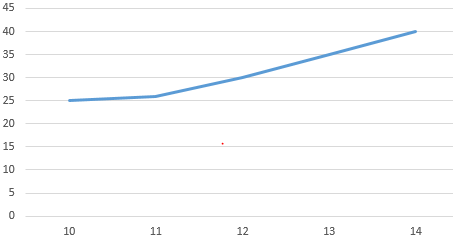## Cartesian system

The system of fixing a point with the help of two measurements, vertical and horizontal is known as Cartesian system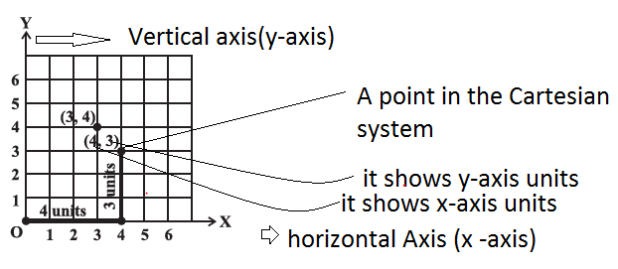Important points
(1) The horizontal axis is called X-axis
(2) The vertical axis is called the Y-axis
(3) O is the origin
(4) Any point is depicted by distance from both the axis
(5) The horizontal distance is called the x-coordinate
(6) The vertical distance is called the y -coordinate
Example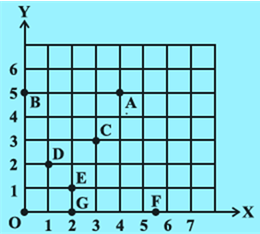Find the coordinates of the each of the point in the given below figure
Solution
A( 4,5)
B(5,0)
C(3,3)
D( 1,2)
E(2,1)
F(5.5,0)
G( 2,0)

(1) __________________ are visual representations of data collected.
(2) A line graph which is a whole unbroken line is called a _____
(3) The system of fixing a point with the help of two measurements, vertical and horizontal is known as _________
(4) Cartesian system is named in honour of 17th century mathematician ______
(5) (0,0) is called _____

## Summary

1. A line graph displays data that changes continuously over periods of time.
2. A line graph which is a whole unbroken line is called a linear graph.
3. For fixing a point on the graph sheet we need, x-coordinate and y-coordinate.
4. The relation between dependent variable and independent variable is shown through a graph